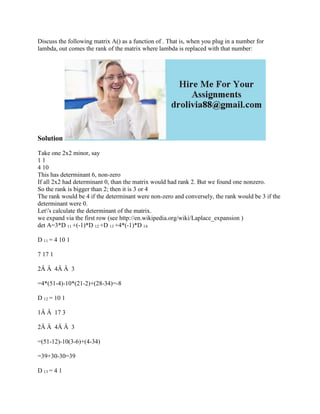Ce diaporama a bien été signalé.
Le téléchargement de votre SlideShare est en cours. ×

# Discuss the following matrix A() as a function of - That is- when you.docx

Publicité
Publicité
Publicité
Publicité
Publicité
Publicité
Publicité
Publicité
Publicité
Publicité
PublicitéChargement dans…3
×

## Consultez-les par la suite

1 sur 2 Publicité

# Discuss the following matrix A() as a function of - That is- when you.docx

Discuss the following matrix A() as a function of . That is, when you plug in a number for lambda, out comes the rank of the matrix where lambda is replaced with that number:
Solution
Take one 2x2 minor, say
1 1
4 10
This has determinant 6, non-zero
If all 2x2 had determinant 0, than the matrix would had rank 2. But we found one nonzero.
So the rank is bigger than 2; then it is 3 or 4
The rank would be 4 if the determinant were non-zero and conversely, the rank would be 3 if the determinant were 0.
Let\'s calculate the determinant of the matrix.
we expand via the first row (see http://en.wikipedia.org/wiki/Laplace_expansion )
det A=3*D 11 +(-1)*D 12 +D 13 +4*(-1)*D 14
D 11 = 4 10 1
7 17 1
2Â Â  4Â Â  3
=4*(51-4)-10*(21-2)+(28-34)=-8
D 12 = 10 1
1Â Â  17 3
2Â Â  4Â Â  3
=(51-12)-10(3-6)+(4-34)
=39+30-30=39
D 13 = 4 1
1 7 3
2Â Â  2 3
=(21-6)-4(3-6)+2-14=15+12-12=15
D 14 = 4 10
1 7Â Â Â  17
2Â Â  2Â Â Â  4
=(28-34)-4(4-34)+10(2-14)=-6
So we have
det A=3*(-8)-39+15-4(-6)=
=-24-39+15+24=-24
The determinant cannot nonzero, so the matrix has rank 4
In other words, no matter what is, the rank is always 4.
.

Discuss the following matrix A() as a function of . That is, when you plug in a number for lambda, out comes the rank of the matrix where lambda is replaced with that number:
Solution
Take one 2x2 minor, say
1 1
4 10
This has determinant 6, non-zero
If all 2x2 had determinant 0, than the matrix would had rank 2. But we found one nonzero.
So the rank is bigger than 2; then it is 3 or 4
The rank would be 4 if the determinant were non-zero and conversely, the rank would be 3 if the determinant were 0.
Let\'s calculate the determinant of the matrix.
we expand via the first row (see http://en.wikipedia.org/wiki/Laplace_expansion )
det A=3*D 11 +(-1)*D 12 +D 13 +4*(-1)*D 14
D 11 = 4 10 1
7 17 1
2Â Â  4Â Â  3
=4*(51-4)-10*(21-2)+(28-34)=-8
D 12 = 10 1
1Â Â  17 3
2Â Â  4Â Â  3
=(51-12)-10(3-6)+(4-34)
=39+30-30=39
D 13 = 4 1
1 7 3
2Â Â  2 3
=(21-6)-4(3-6)+2-14=15+12-12=15
D 14 = 4 10
1 7Â Â Â  17
2Â Â  2Â Â Â  4
=(28-34)-4(4-34)+10(2-14)=-6
So we have
det A=3*(-8)-39+15-4(-6)=
=-24-39+15+24=-24
The determinant cannot nonzero, so the matrix has rank 4
In other words, no matter what is, the rank is always 4.
.

Publicité
Publicité

### Discuss the following matrix A() as a function of - That is- when you.docx

1. 1. Discuss the following matrix A() as a function of . That is, when you plug in a number for lambda, out comes the rank of the matrix where lambda is replaced with that number: Solution Take one 2x2 minor, say 1 1 4 10 This has determinant 6, non-zero If all 2x2 had determinant 0, than the matrix would had rank 2. But we found one nonzero. So the rank is bigger than 2; then it is 3 or 4 The rank would be 4 if the determinant were non-zero and conversely, the rank would be 3 if the determinant were 0. Let's calculate the determinant of the matrix. we expand via the first row (see http://en.wikipedia.org/wiki/Laplace_expansion ) det A=3*D 11 +(-1)*D 12 +D 13 +4*(-1)*D 14 D 11 = 4 10 1 7 17 1 2Â Â 4Â Â 3 =4*(51-4)-10*(21-2)+(28-34)=-8 D 12 = 10 1 1Â Â 17 3 2Â Â 4Â Â 3 =(51-12)-10(3-6)+(4-34) =39+30-30=39 D 13 = 4 1
2. 2. 1 7 3 2Â Â 2 3 =(21-6)-4(3-6)+2-14=15+12-12=15 D 14 = 4 10 1 7Â Â Â 17 2Â Â 2Â Â Â 4 =(28-34)-4(4-34)+10(2-14)=-6 So we have det A=3*(-8)-39+15-4(-6)= =-24-39+15+24=-24 The determinant cannot nonzero, so the matrix has rank 4 In other words, no matter what is, the rank is always 4.